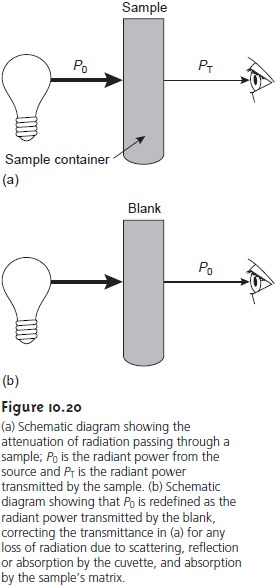Home | | Modern Analytical Chemistry | Transmittance and Absorbance - Spectroscopy Based on Absorption

# Transmittance and Absorbance - Spectroscopy Based on AbsorptionThe attenuation of electromagnetic radiation as it passes through a sample is described quantitatively by two separate, but related terms: transmittance and absorbance.

Transmittance and Absorbance

The attenuation of electromagnetic radiation as it passes through a sample is described quantitatively by two separate, but related terms: transmittance and absorbance. Transmittance is defined as the ratio of the electromagnetic radi- ation’s power exiting the sample, PT, to that incident on the sample from the source, P0, (Figure 10.20a).10.1

Multiplying the transmittance by 100 gives the percent transmittance (%T), which varies between 100% (no absorption) and 0% (complete absorption). All methods of detection, whether the human eye or a modern photoelectric transducer, measure the transmittance of electromagnetic radiation.

Attenuation of radiation as it passes through the sample leads to a trans- mittance of less than 1. As described, equation 10.1 does not distinguish be- tween the different ways in which the attenuation of radiation occurs. Besides absorption by the analyte, several additional phenomena contribute to the net attenuation of radiation, including reflection and absorption by the sample container, absorption by components of the sample matrix other than the an- alyte, and the scattering of radiation. To compensate for this loss of the elec- tromagnetic radiation’s power, we use a method blank (Figure 10.20b). The radiation’s power exiting from the method blank is taken to be P0.

An alternative method for expressing the attenuation of electromagnetic radiation is absorbance, A, which is defined as10.2

Absorbance is the more common unit for expressing the attenuation of radiation because, as shown in the next section, it is a linear function of the analyte’s concentration.Equation 10.1 has an important consequence for atomic absorption. Because of the narrow line width for atomic absorption, a continuum source of radiation can- not be used. Even with a high-quality monochromator, the effective bandwidth for a continuum source is 100–1000 times greater than that for an atomic absorption line. As a result, little of the radiation from a continuum source is absorbed (Po = PT), and the measured absorbance is effectively zero. For this reason, atomic ab- sorption requires a line source.

Study Material, Lecturing Notes, Assignment, Reference, Wiki description explanation, brief detail
Modern Analytical Chemistry: Spectroscopic Methods of Analysis : Transmittance and Absorbance - Spectroscopy Based on Absorption |